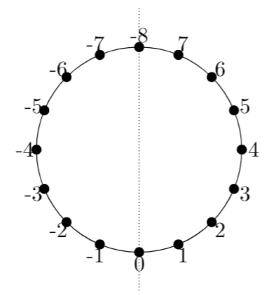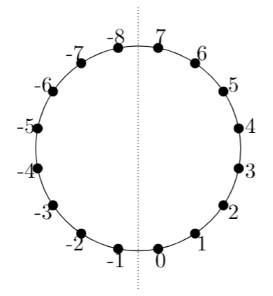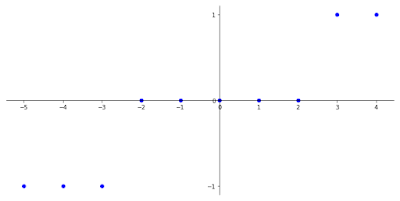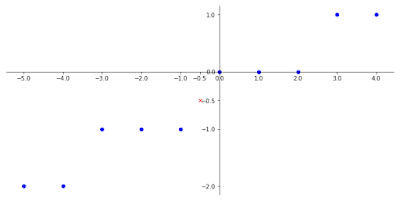## Wednesday, 3 October 2018

### abs and its "extra" result

The abs function has, in its usual (most useful) formulation, one extra value in its codomain than just "all non-negative values". That extra value is the most negative integer, which satisfies abs(x) == x despite being negative. Even accepting that the absolute value of the most negative integer is itself, it may still seem strange (for an operation that is supposed to have such a nice symmetry) that the size of the codomain is not exactly half of the size of the domain.

That there is an "extra" value in the codomain, and that it is specifically the most negative integer, may be more intuitively obvious when the action of abs on the number line circle is depicted as "folding" the circle symmetrically in half across the center and through zero (around which abs is supposed to be symmetric), folding the negative numbers onto the corresponding positive numbers:Clearly both zero and the most negative integer (which is also on the "folding line") stay in place in such a folding operation and remain part of the resulting half-circle. That there is an "extra" value in the codomain is the usual fencepost effect: the resulting half-circle is half the size of the original circle in some sense, but the "folding line" cuts through two points that have now become endpoints.

By the way the "ones' complement alternative" to the usual abs, let's call it OnesAbs(x) = x < 0 ? ~x : x (there is a nice branch-free formulation too) does have a codomain with a size exactly half of the size of its domain. The possible results are exactly the non-negative values. It has to pay for that by, well, not being the usual abs. The "folding line" for OnesAbs runs between points, avoiding the fencepost issue:## Tuesday, 21 August 2018

### Signed wrapping is meaningful and algebraically nice

In this post I defend wrapping, a bit more opinionated than my other posts. As usual I'm writing from the perspective that signed and unsigned integer types are a thin transparent wrapper around bit vectors, of course I am aware that they are often not used that way. That difference between their use and their actual nature is probably the source of the problems.

## Signed wrapping is not wrong

It is often said that when signed wraparound occurs, the result is simply wrong. That is an especially narrow view to take, probably inspired by treating fixed-size bit vector arithmetic as if it is arithmetic in ℤ, which it is not. Bit vector arithmetic can be viewed as arithmetic in ℤ so long as no "overflow" occurs, but violating that condition does not make the result wrong, it makes the interpretation wrong.

## Signed wrapping is meaningful

The wrapping works exactly the same as unsigned wrapping, it corresponds to taking the lowest k bits of the arbitrary precision result. Such a truncation therefore gives you exactly k meaningful bits, it's just a slice of the result. Some upper bits may be lost, they can be calculated if you need them. If the whole result is meaningful, then part of it is too, namely at least under the interpretation of being "part of the result".

An other well known example of benign wrapping is the calculation of the average of two non-negative signed integers. While (a + b) / 2 gives inconvenient results when the addition "overflows", (uint)(a + b) / 2 (using unsigned division) or (a + b) >>> 1 (unsigned right shift as in Java) are correct even when the addition of two positive values results in a negative value. An other way to look at it is that there is no unsigned wrapping. Nominally the integers being added here are signed but that doesn't really matter. Casting the inputs to unsigned before adding them is a no-op that can be performed mentally.

Wrapping can also sometimes be cancelled with more wrapping. For example, taking an absolute value with wrapping and casting the result to an unsigned type of the same width results in the actual absolute value without the funny int.MinValue edge case:

(uint)abs(int.MinValue) =
(uint)abs(-2147483648) =
(uint)(-2147483648) =
2147483648

This is not what Math.Abs in C# does, it throws, perhaps inspired by its signed return type. On the other hand, Java's Math.abs gets this right and leaves the reinterpretation up to the consumer of the result, of course in Java there is no uint32 to cast to but you can still treat that result as if it is unsigned. Such "manual reinterpretation" is in general central to integer arithmetic, it's really about the bits, not the "default meaning" of those bits.

The principle of cancelling wrapping also has some interesting data structure applications. For example, in a Fenwick tree or Summed Area Table, the required internal integer width is the desired integer width of any range/area-sum query that you actually want to make. So a SAT over signed bytes can use an internal width of 16 bits as long as you restrict queries to an area of 256 cells or fewer, since 256 * -128 = -215 which still fits a signed 16 bit word.

An other nice case of cancelled wrapping is strength reductions like A * 255 = (A << 8) - A. It is usually not necessary to do that manually, but that's not the point, the point is that the wrapping is not "destructive". The overall expression wraps only iff A * 255 wraps and even then it has exactly the same result. There are cases in which the left shift experience "signed wrapping" but A * 255 does not (for example, in 32 bits, A = 0x00800000), in those cases the subtraction also wraps and brings the result back to being "unwrapped". That is not a coincidence nor an instance of two wrongs making a right, it's a result of the intermediate wrapped result being meaningful and wrapping being algebraically nice.

## Signed wrapping is not inherent

Signed and unsigned integers are two different ways to interpret bit vectors. Almost all operations have no specific signed or unsigned version, only a generic version that does both. There is no such thing as signed addition or unsigned addition, addition is just addition. Operations that are actually different are:

• Comparisons except equality
• Division and remainder
• Right shift, maybe, but arithmetic right shift and logical right shift can both be reasonably applied in both signed and unsigned contexts
• Widening conversion
• Widening multiplication
One thing almost all of these have in common is that they cannot overflow, except division of the smallest integer by negative one. By the way I regard that particular quirk of division as a mistake since it introduces an asymmetry between dividing by negative one and multiplying by negative one.

The result is that the operations that can "overflow" are neither signed nor unsigned, and therefore do not overflow specifically in either of those ways. If they can be said to overflow at all, when and how they do so depends on how they are being viewed by an outside entity, not on the operation itself.

The distinction between unsigned and signed wrapping is equivalent to imagining a "border" on the ring of integers (not the mathematical Ring of Integers) either between 0 and -1 (unsigned) or between signed-smallest and signed-highest numbers, but there is no border. Crossing either of the imaginary borders does not mean nearly as much as many people think it means.

## Signed wrapping is algebraically nice

A property that wrapping arithmetic shares with arbitrary precision integer arithmetic, but not with trapping arithmetic, is that it obeys a good number of desirable algebraic laws. The root cause of this is that ℤ/ℤ2k is a ring, and trapping arithmetic is infested with implicit conditional exceptions. Signed arithmetic can largely be described by ℤ/ℤ2k, like unsigned arithmetic, since it is mostly a reinterpretation of unsigned arithmetic. That description does not cover all operations or properties, but it covers the most important aspects.

Here is a small selection of laws that apply to wrapping arithmetic but not to trapping arithmetic:

• -(-A) = A
• A + -A = 0
• A - B = A + -B
• A + (B + C) = (A + B) + C
• A * (B + C) = A * B + A * C
• A * -B = -A * B = -(A * B)
• A * (B * C) = (A * B) * C
• A * 15 = A * 16 - A
• A * multiplicative_inverse(A) = 1 (iff A is odd, this is something not found in ℤ which has only two trivially invertible numbers, so sometimes wrapping gives you a new useful property)
Some laws also apply to trapping arithmetic:
• A + 0 = A
• A - A = 0
• A * 0 = 0
• A * 1 = A
• A * -1 = -A
• -(-(-A)) = -A

The presence of all the implicit exceptional control flow makes the code very hard to reason about, for humans as well as compilers.

Compilers react to that by not optimizing as much as they otherwise would, since they are forced to preserve the exception behaviour. Almost anything written in the source code must actually happen, and in the same order as originally written, just to preserve exceptions that are not even supposed to ever actually be triggered. The consequences of that are often seen in Swift, where code using the &+ operator is optimized quite well (including auto-vectorization) and code using the unadorned + operator can be noticeably slower.

Humans .. probably don't truly want trapping arithmetic to begin with, what they want is to have their code checked for unintended wrapping. Wrapping is not a bug by itself, but unintended wrapping is. So while canceling a "bare" double negation is not algebraically justified in trapping arithmetic, a programmer will do it anyway since the goal is not to do trapping arithmetic, but removing bad edge cases. Statically checking for unintended wrapping would be a more complete solution, no longer relying on being lucky enough to dynamically encounter every edge case. Arbitrary precision integers would just remove most edge cases altogether, though it would rely heavily on range propagation for performance, making it a bit fragile.

But anyway, wrapping is not so bad. Just often unintended.

## Thursday, 2 August 2018

### Implementing Euclidean division

While implementing various kinds of division in haroldbot, I had to look up/work out how to implement different kinds of signed division in terms of unsigned division. The common truncated division (written as /s in this post and in haroldbot, /t in some other places) is natural result of using your intuition from ℝ and writing the definition based on signs and absolute values, ensuring that the division only happens between non-negative numbers (making its meaning unambiguous) and that the result is an integer: $$\DeclareMathOperator{\sign}{sign} D /_s d = \sign(d)\cdot\sign(D)\cdot\left\lfloor\cfrac{\left|D\right|}{\left|d\right|}\right\rfloor$$ That definition leads to a plot like this, showing division by 3 as an example:Of course the absolute values and sign functions create symmetry around the origin, and that seems like a reasonable symmetry to have. But that little plateau around the origin often makes the mirror at the origin a kind of barrier that you can run into, leading to the well-documented downsides of truncated division.

The alternative floored division and Euclidean division have a different symmetry, which does not lead to that little plateau, instead the staircase pattern simply continues:The point of symmetry, marked by the red cross, is at (-0.5, -0.5). Flipping around -0.5 may remind you of bitwise complement, especially if you have read my earlier post visualizing addition, subtraction and bitwise complement, and mirroring around -0.5 is no more than a conditional complement. So Euclidean division may be implemented with positive division as: $$\DeclareMathOperator{\sgn}{sgn} \DeclareMathOperator{\xor}{\bigoplus} D /_e d = \sign(d)\cdot(\sgn(D)\xor\left\lfloor\cfrac{D\xor\sgn(D)}{\left|d\right|}\right\rfloor)$$ Where the sgn function is -1 for negative numbers and 0 otherwise, and the circled plus is XOR. XORing with the sgn is a conditional complement, with the inner XOR being responsible for the horizontal component of the symmetry and the outer XOR being responsible for the vertical component.

It would have been even nicer if the symmetry of the divisor also worked that way, but unfortunately that doesn't quite work out. For the divisor, the offset introduced by mirroring around -0.5 would affect the size of the steps of the staircase instead of just their position.

The /e and %e operators are available in haroldbot, though like all forms of division the general case is really too hard, even for the circuit-SAT based truth checker (the BDD engine stands no chance at all).